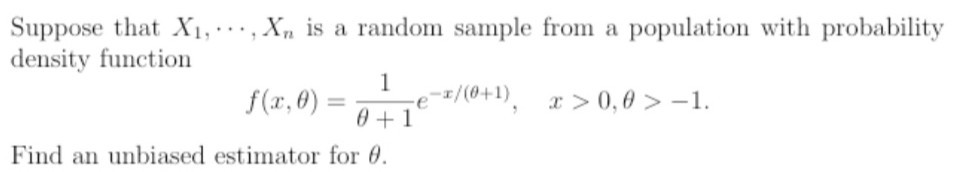Home / Answered Questions / Other / suppose-that-x1-x-is-a-random-sample-from-a-population-with-probability-density-function-f-x-0-1-0-1-aw206

# (Solved): Suppose That X1,..., X, Is A Random Sample From A Population With Probability Density Function F(x,0...Suppose that X1,..., X, is a random sample from a population with probability density function f(x,0) = -1/(0+1), 2>0,0 > -1. 0+1 Find an unbiased estimator for 0.

We have an Answer from Expert